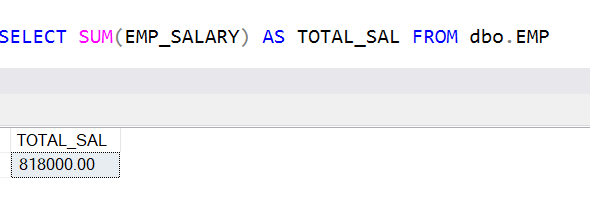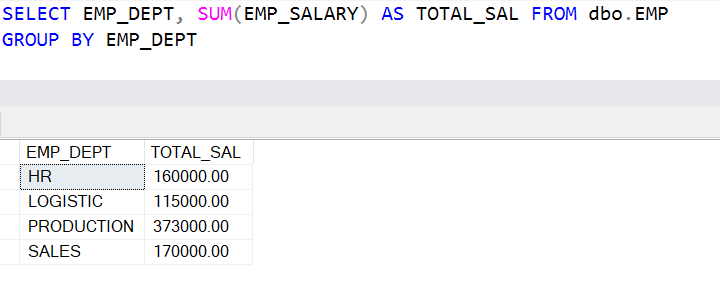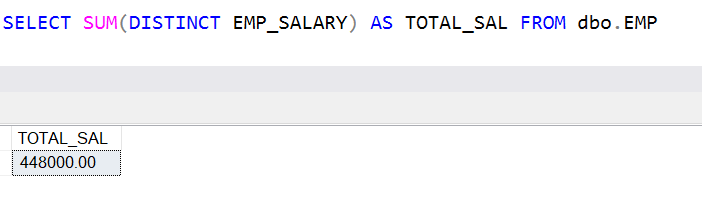Home » SQL Server SUM

# SQL Server SUMSQL Server SUM function is an aggregate that returns the sum of values for any column in a table.

SYNTAX

`SUM(expression)  `

Lets look at an example of SUM function in SQL Server.

First we create a sample table named as EMP and insert some records into this table as shown below.

```CREATE TABLE dbo.EMP (
EMPID INT NOT NULL,
EMP_DEPT VARCHAR(50),
EMP_NAME VARCHAR(50),
EMP_SALARY NUMERIC(9,2)
)

INSERT INTO dbo.EMP
(EMPID, EMP_DEPT, EMP_NAME, EMP_SALARY)
VALUES
(101, 'PRODUCTION', 'RAJAT M',75000.00),
(102, 'PRODUCTION', 'MUKESH BHATIA',70000.00),
(103, 'PRODUCTION', 'MUSKAN MEHTA',75000.00),
(104, 'SALES', 'ROHAN B NARAYA',45000.00),
(105, 'SALES', 'SUSHIL DAS',40000.00),
(106, 'SALES', 'MANISH',45000.00),
(107, 'PRODUCTION', 'RAJESH SINGH',78000.00),
(108, 'HR', 'MOHIN KHAN',50000.00),
(109, 'HR', 'SUSHANT K SINGH',55000.00),
(110, 'HR', 'LAKSHYA RAWAT',55000.00),
(111, 'PRODUCTION', 'MANOJ KUMAR',75000.00),
(112, 'SALES', 'SUJOY M',40000.00),
(113, 'LOGISTIC', 'VINAY AGARWAL',35000.00),
(114, 'LOGISTIC','MUSTAKIM M',35000.00),
(115, 'LOGISTIC', 'VIJAY KUMAWAT',45000.00)```

#### Get the total salary of all employee’s

Following statement returns the total salary of all employees in table EMP.

`SELECT SUM(EMP_SALARY) AS TOTAL_SAL FROM dbo.EMP`#### Get the total salary of all employees by department

Following statement returns the total salary of employees by department.

```SELECT EMP_DEPT, SUM(EMP_SALARY) AS TOTAL_SAL FROM dbo.EMP
GROUP BY EMP_DEPT```#### Get the total salary of employees for PRODUCTION DEPARMENT

Following statement returns the total salary for production department.

```SELECT EMP_DEPT, SUM(EMP_SALARY) AS TOTAL_SAL FROM dbo.EMP
WHERE  EMP_DEPT ='PRODUCTION'
GROUP BY EMP_DEPT```#### Get the department by total salary more than 150000

Following statement returns the total salary greater than 150000 by department.

```SELECT EMP_DEPT, SUM(EMP_SALARY) AS TOTAL_SAL FROM dbo.EMP
GROUP BY EMP_DEPT
HAVING SUM(EMP_SALARY)> 150000```#### Get the sum of unique salaries in the EMP table

Following statement returns the sum of unique salaries.You can also see the other Aggregate functions.

SQL AVG Function

SQL COUNT Function

SQL MIN Function

SQL MAX Function

1,836 total views,  1 views today

This site uses Akismet to reduce spam. Learn how your comment data is processed.